# Texas Go Math Grade 5 Lesson 14.4 Answer Key Graph and Analyze Relationships

Refer to our Texas Go Math Grade 5 Answer Key Pdf to score good marks in the exams. Test yourself by practicing the problems from Texas Go Math Grade 5 Lesson 14.4 Answer Key Graph and Analyze Relationships.

## Texas Go Math Grade 5 Lesson 14.4 Answer Key Graph and Analyze Relationships

Unlock the Problem

Sasha is making hot cocoa for a party. For each mug of cocoa, he uses 3 tablespoons of cocoa mix. If Sasha makes 9 mugs of cocoa, how many tablespoons of the cocoa mix will he use?

Step 1
Use the rule to make an input/output table.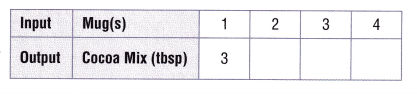Step 2
Write the related pairs of data as ordered pairs.
(1, 3) ___ ___ ____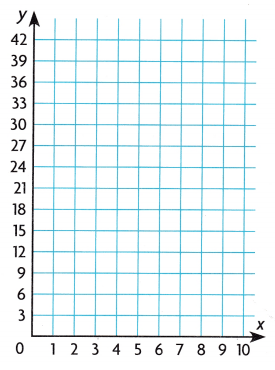Step 3
Plot and label the ordered pairs in the coordinate grid. Use the pattern to write an ordered pair for the number of tablespoons of the cocoa mix needed for 9 mugs of cocoa. Plot the point in the coordinate grid.
So, Sasha will need ___ tablespoons of the cocoa mix to make 9 mugs of cocoa.

• How can you use the pattern in the coordinate grid to decide if your answer is reasonable?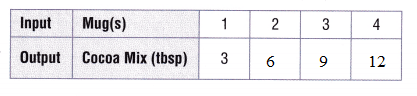(1, 3) (2, 6) (3, 9) (4, 12)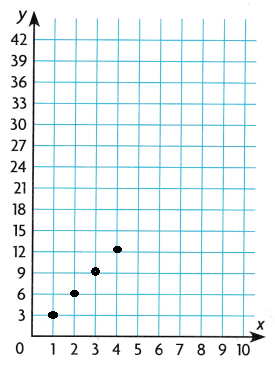Share and Show

Complete the input/output tables. Write ordered pairs and plot them in the coordinate grid.

Question 1.
Multiply the number of tablespoons by 2 to find the weight in ounces.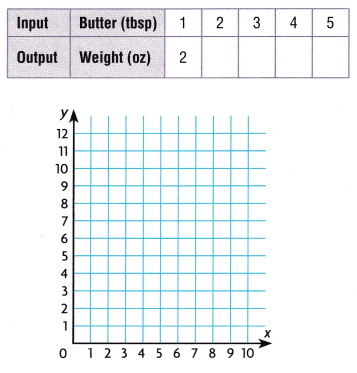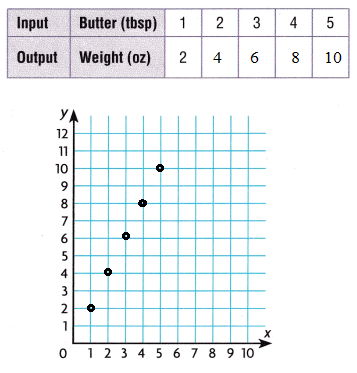Question 2.
Multiply the number of hours by 3 to find the distance in miles.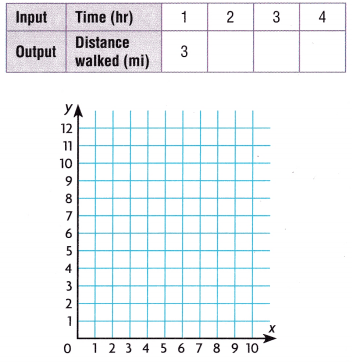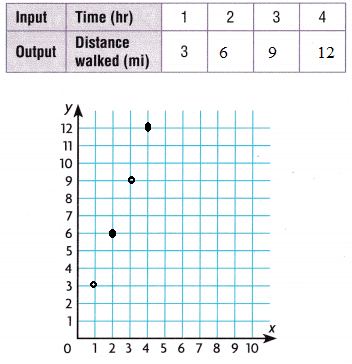Problem Solving

Use the graph for 3-4.

Question 3.
H.O.T. Multi-Step The rule for the pattern is multiply the input by 5. Which ordered pair on the graph 80
does not follow the pattern? Explain.(3, 20). The input is 3, so the output is 5 × 3 or 15. The ordered pair would be (3, 15), not (3, 20)

Question 4.
H.O.T. Communicate If the input is 12, would the output be greater or less than 40? Write the ordered pair and plot it on the graph.
Greater than 40. (12, 60) when the input is 8, the ouput is 40. So, if the input is 12, the output would be greater than 40. when the input is 12 5 × 12 = 60.

Problem Solving

Use the coordinate grid for 5-6. Complete the table for each recipe and plot the points. Use different colors to plot each person’s pattern.

Question 5.
Lou and George are making chili for the Annual Firefighter’s Ball.
Lou uses 2 teaspoons of hot sauce for every 2 cups of chili. 1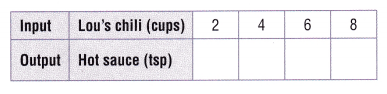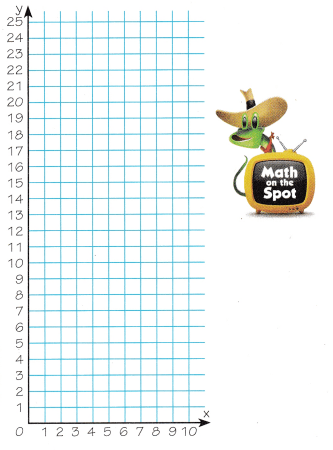George uses 3 teaspoons of the same hot sauce for every cup of chili.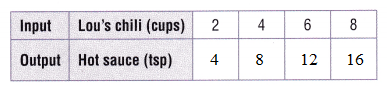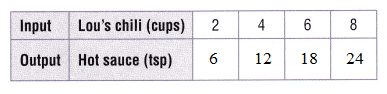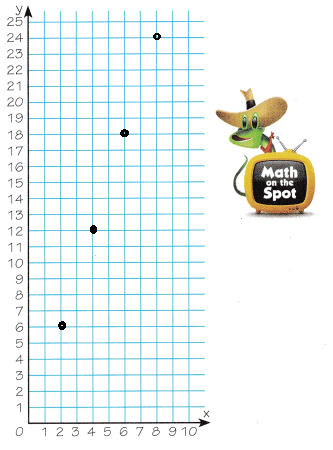Question 6.
Sense or Nonsense? Elsa said that George’s chili was hotter than Lou’s, because the graph showed that the amount of hot sauce in George’s chili was always 3 times as great as the amount of hot sauce in Lou’s chili. Does Elsa’s answer make sense, or is it nonsense? Explain.
Elsa’s makes sense. I can see on the graph that for the same x-coordinate the y-coordinate is 3 times greater for George’s chili then Lou’s chili. So, George’s chili is 3 times as hot.

Question 7.
H.O.T. Multi-Step If you mix 10 cups of George’s chili with 10 cups of Lou’s chili, how many teaspoons of hot sauce will there be in that 20 cups of chili?
40 tsp

Question 8.
The table compares distance on a map to real-life distance. How many miles does a map distance of 6 inches represent ?(A) 24 miles
(B) 30 miles
(C) 36 miles
(D) 6 miles

Use the graph for 9-10.

Question 9.
Which statement about the data is correct?
(A) One pen costs $5. (B) Four pens cost$20.
(C) Two pens cost S5.
(D) Five pens cost$1.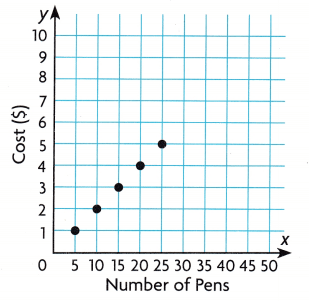Answer: (D) Five pens cost$1.

Question 10.
Multi-Step Suppose Jake buys 30 pens. He also buys a notebook for $3. How much does lake spend in all? (A)$6
(B) $9 (C)$33
(D) $30 Answer: (B)$9

Texas Test Prep

Question 11.
Duber plots a pattern showing the number of pentagons and the total number of sides for that many pentagons. If the x-coordinate, the number of pentagons, is 8, which ordered pair shows the pattern?
(A) (8, 5)
(B) (5, 8)
(C) (40, 8)
(D) (8, 40)

### Texas Go Math Grade 5 Lesson 14.4 Homework and Practice Answer Key

Complete the input/output tables. Write ordered pairs and plot them in the coordinate grid.

Question 1.
Multiply the number of days by 5 to find the number of hours worked.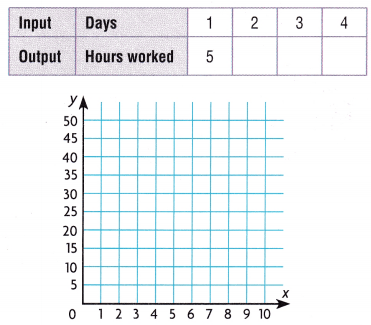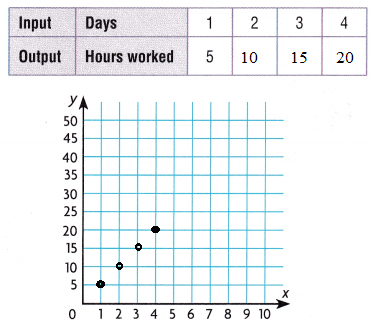Question 2.
Multiply the number of gallons by 4 to find the number of quarts.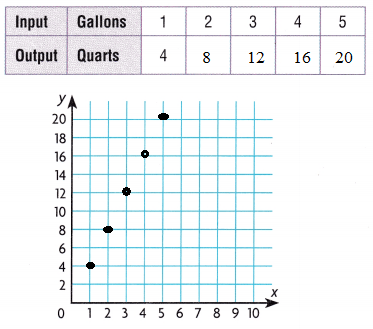Problem Solving

Use the coordinate grid for 3-4. Complete the table for each person and plot the points. Use different colors to plot each person’s pattern.

Question 3.
Marion uses 2 buttons for each doll.Nola uses 4 buttons for each doll.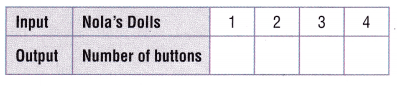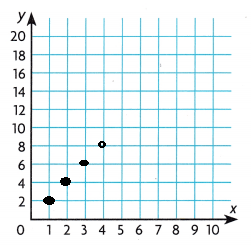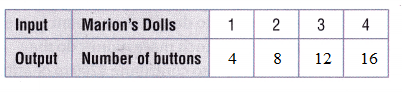Question 4.
How many buttons do Marion and Nola use altogether if they each make 5 dolls?

Lesson Check

Question 5.
Shane plots a pattern on a graph that shows the relationship for length of one side of a square and the area of the square. If the x-coordinate, the length of one side of a square, is 6 inches, which ordered pair will Shane plot?
(A) (6, 24)
(B) (6, 36)
(C) (36, 6)
(D) (6, 6)

Question 6.
The table compares distance on a map to actual distance. How many kilometers does a map distance of 8 centimeters represent?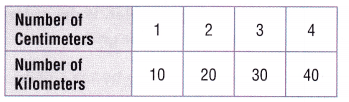(A) 50 kilometers
(B) 8 kilometers
(C) 40 kilometers
(D) 80 kilometers

Use the graph for 7-9.

Question 7.
Which statement about the data is correct?
(A) The amount earned for washing one car is $10. (B) The amount earned for washing 20 cars is one dollar per car. (C) The amount earned for washing one car is$20.
(D) The amount earned for washing 10 cars is $50.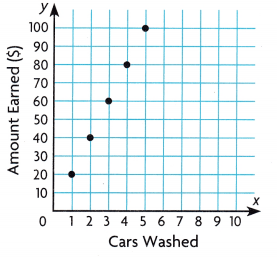Answer: (C) The amount earned for washing one car is$20.

Question 8.
Multi-Step Suppose LeAnn washes 2 cars on Friday and 3 cars on Saturday. How much does she earn?
(A) $40 (B)$60
(C) $50 (D)$100
Answer: (D) $100 Explanation: The amount earned for washing one car is$20.
2 cars = 2 × $20 =$40
3 cars = 3 × $20 =$60
$40 +$60 = $100 Question 9. Multi-Step Kyle washes 4 cars. Erin washes 5 cars. Both plan to donate the amount earned to charity. How much more money do they need if they want to contribute$200 to charity?
(A) $20 (B)$180
(C) $40 (D)$100
The amount earned for washing one car is $20. 4 cars = 4 ×$20 = $80 5 cars = 5 ×$20 = $100$200 – $180 =$20